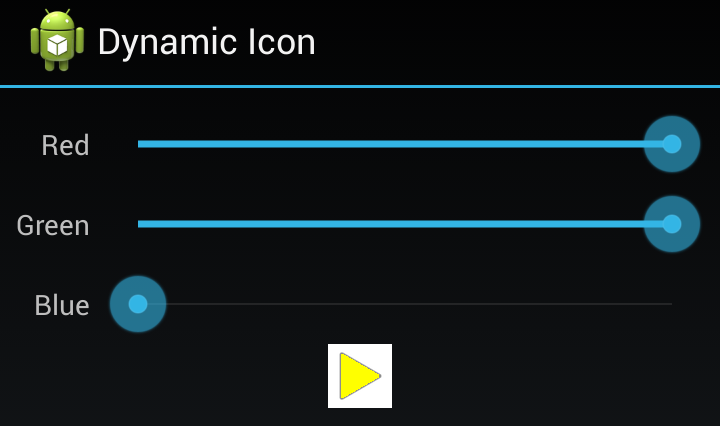# Dynamic Icon – Part 2

In the previous article we got a basic app set up and got our images set up ready to create an image in which part of the colour could change depending on the values of three SeekBars representing the red, green, and blue components. In this article we’ll look at how we actually apply those colour values and create the required image.

Previously we got everything written with the exception of an method named `updateImage()`, so let’s look at that:

There’s not actually much code there, but it requires a little bit of explanation. If we focus on `combineImages` to start with, what we are doing is creating a new Bitmap which is maximum width and height of our background and foreground images. Then we’re creating a Canvas based upon that Bitmap. Then we draw our background image on the Canvas at position 0,0 and we then draw the foreground image on top of this at position 0,0 using a Paint object on which we’ve set a ColorFilter. We’ll come back to the ColorFilter in a moment but for now let’s just assume that it applies the appropriate colour as we draw the foreground image.

The Bitmap containing this combined image can then be applied to our ImageView object. So the combining of the images is pretty straightforward, but this ColorFilter requires some further explanation.

If we look at the creation of the ColorFilter it looks pretty scary. The constructor takes an array of 20 float values:

It becomes a little less daunting if we break this down in to 4 groups of 5 floats. Each group corresponds to one of the 4 colour channels: red, green, blue, and alpha (transparency). Each of the values in each group represents part of the calculation to determine the new value for that colour channel for that pixel.

Let’s break it down a little further. If we have the following matrix:

```{ a, b, c, d, e, f, g, h, i, j, k, l, m, n, o, p, q, r, s, t } ```

and we apply this to an arbitrary colour represented by four values to represent the red, green, blue, and alpha components:

`[ R, G, B, A ]`

The resulting colour will be made up of four new red, green, blue, and alpha components. Each of these is based upon a quite simple calculation. Let’s consider the new red value first, which is calculated as follows:

`R' = R*a + G*b + B*c + A*d + e`

So the new red value can be influenced by *any* of the existing colour channel values, which can be really useful for doing things like inverting the colours of an image. In our case, we use the following values:

```a = red (the value from our red SeekBar) b = 0 c = 0 d = 0 e = 0```

So the calculation can be simplified to:

```R' = R*a G' = G*g B' = B*m```

So if we think about our mask image, all of the pixels were either white or transparent. When the matrix is applied to a white pixel (which has 0xFF red component) from the mask, the new red value will be used. The green and blue values will be calculated in the same way, and for this reason it is important that the “on” pixels in our mask are white because the output values will depend on the green and blue components of the mask pixel.

But what about those transparent pixels from the mask, how does that work? When the transform applied to the alpha channel the alpha channel part of the matrix will be used:

```p = 0 q = 0 r = 0 s = 1.0 t = 0```

So the calculation is:

`A' = A*s`

This is actually an identity transformation where the value output will match the value input. So in our case where the mask pixel is transparent, the output will be transparent (i.e. the background image will show through) and where the mask pixel is opaque the foreground image will overwrite the background image.

For the sake of clarity, our mask only contains fully opaque or fully transparent pixels. However by using varying levels of transparency we can allow parts of the background image to show through.

If we look at what this does to our app we can see that our colour is now applied to the image:

Specifying a light colour demonstrates that the border of the background image is showing through our colourised mask:That concludes this short series of articles, but we’ve barely scratched the surface of what a colour matrix transform can do but, suffice it to say, they are extremely powerful!

Information about how to reuse or republish this work may be available at http://blog.stylingandroid.com/license-information.

## About Mark Allison1.Mark Allison says: